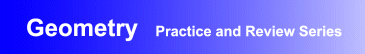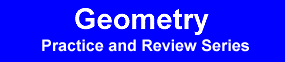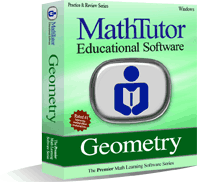Award-Winning

# Geometry Interactive Review and Practice SoftwareDESCRIPTION
GEOMETRY is a top rated software package designed to help students build problem solving skills while reinforcing their understanding of basic concepts and proofs.
Geometry drill and practice exercises move from basic problems to the more challenging, allowing students to develop problem solving skills at a comfortable pace. Videos and dynamic animations provide step by step explanations.
GEOMETRY is one of the titles in the Practice and Review Series, the most comprehensive drill and practice software available.
Students are continually motivated by fresh problems, tailored to their abilities and generated by randomization techniques. Particular attention is devoted to helping students develop skills in preparing proofs, which many students have difficulty with.
• Geometry drill and practice software developed by math curriculum specialists
• Interactive geometry exercises that allow students to develop problem solving skills gradually
• Proven effectiveness helping students master geometry problem solvingTOPICS COVERED
Fundamental Material
• Definitions
• Theorems and Formulas
• Converses
• Constructions
Review of Arithmetic
• Solving an Algebraic Equation
• Fractions: Comparisons
• Fractions: Qualitative
• Arithmetic Comparisons
• Addition and Subtraction of Angles
• Addition and Subtraction of Line Segments
• Angles: Correct Conclusions
• Angles: Incorrect Conclusions
Figures Involving Triangles
• Isosceles Triangles
• Angles About a Triangle
• Congruent Triangles
• Congruent Angles
• Angle Calculations
• Parallel Lines
• Indirect Proof
• Inequalities in a Triangle
Figures Involving Parallels
• Proportional Lengths
• Angle Comparisons
• Parallelograms
• Coordinates in a Rectangle
• Trapezoids and Right Triangles
• Triangles (connect midpoints); Angles
• Rectangles and Angles
Figures Involving Circles and Polygons
• Proportion: Area, Length, Volume
• Arcs and Angles: Triangle in a Circle
• Arcs and Angles: Circle in a Triangle
• Area Between Two Figures
• Triangles: Midpoints and Areas
• Tangents and Secants
• Historical Summary